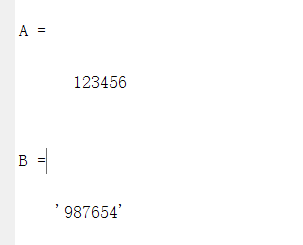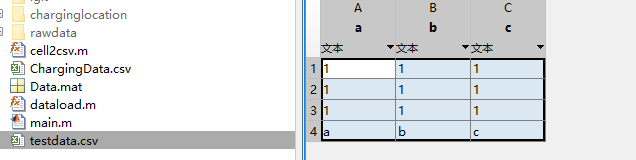• 将数字转换为字符串： num2str（） 将字符串转换为数字： ...A = str2num(a) % 将字符串转化为数字（也可用 A = str2double(a) 对标量进行操作） B = num2str(b) % 将数字转化为字符串 输出结果 ...

将数字转换为字符串：

 num2str（）


将字符串转换为数字：

 str2num（）


示例：

a = '123456';
b = 987654;
A = str2num(a)  % 将字符串转化为数字（也可用 A = str2double(a) 对标量进行操作）
B = num2str(b)  % 将数字转化为字符串

输出结果展开全文• matlab的字符串拼接本质上是字符数组的拼接，和通常数组的拼接方法一致，都是使用 [] 与 空格 进行拼接的，如： >> a = [1 2 3];b = [4 5 6 7]; >> c = [a b] c = ...首先字符串转化为...
matlab的字符串拼接本质上是字符数组的拼接，和通常数组的拼接方法一致，都是使用 [] 与 空格 进行拼接的，如：
>> a = [1 2 3];b = [4 5 6 7];
>> c = [a b]
c =
1     2     3     4     5     6     7

所以问题的关键在于如何把各种字符串、数字等转化为字符数组。
首先字符串转化为字符数组使用 char(str) 函数，如：
>> char("abcd")
ans =
'abcd'

在这里我们看到一个关键问题，双引号与单引号的区别，双引号是字符串，单引号是字符数组。
于是拼接字符串代码如下：
>> ['hello' ' ' 'world']
ans =
'hello world'

或者你也可以写成这样：
>> [char("hello") ' ' char("world")]
ans =
'hello world'

如果直接用双引号会是这样：
>> ["hello" " " "world"]
ans =
1×3 string 数组
"hello"    " "    "world"

同样的，加入数字的字符串拼接如下：
>> for i=1:3
disp(['data6/DATA' char(num2str(i)) '.TXT']);
end
data6/DATA1.TXT
data6/DATA2.TXT
data6/DATA3.TXT



展开全文• MATLAB中，有一些对于字符串操作的函数，通过这些函数可以使对于字符串的操作十分简单，现在，就来介绍一些对于字符串的基本操作： 建立一个字符串向量，然后对该向量做如下处理： （1）取 2~6 个字符组成子字符...
在MATLAB中，有一些对于字符串操作的函数，通过这些函数可以使对于字符串的操作十分简单，现在，就来介绍一些对于字符串的基本操作：
建立一个字符串向量，然后对该向量做如下处理：
（1）取 2~6 个字符组成子字符串。
（2）分别取第偶数个字符组成子字符串。
（3）取出里面的数字并转化为数值，然后求和。
（4）去掉字符串中的数字字符。
（5）将字符串按照倒序重新排列。
（6）将字符串中的小写字母变成大写字母，其他不变。
（7）统计字符串中消息字母的个数

首先创建一个字符串，用变量str存储，并输出，代码如下：
string='lin12g13o1343n53153g154x6i547a  548o645s865h9e56ng';
disp(string);

随机提取若干字符，并组成新的字符：
str1=string(3:8);
disp(str1);

提取偶数个字符，并组成一个新的字符串，首先求解出该字符串的长度，存在变量str_long中，然后从字符串的第二个开始取，间隔步长为2，直至最后。
str_long=length(string);
str2=string(2:2:str_long);
disp(str2);

首先提取字符串中所有的数字，然后用str2num()函数将数字由字符型转换成数值型，接着进行求和。
sum=0;
n=0;
for i=1:str_long
if string(1,i) > '0' & string(1,i) < '9'
num_str=string(1,i);
%          disp(num_str);
n=n+1;
sum=sum+str2num(num_str);
end
end
disp('sum of number');
disp(sum);

首先做出判断，找出所有的字符，并存在一个新的字符串里面，最后用strrep(),函数去除字符串里面所有的空格。
x=1;
for i=1:str_long
if (string(1,i)-'a'>=0 & string(1,i)-'a'< 26)||(string(1,i)-'A'>=0 & string(1,i)-'A'< 26)
str_str(1,x)=string(1,i);
x=x+1;
end
end
str_str=strrep(str_str,' ','');
disp(str_str);

倒序输出字符串：
unstring=string(end:-1:1);
disp(unstring);

upper()函数将字母由小写转换为大写，lower()函数将字母由大写转换为小写，然后其余不是26个英文字母的，按原格式输出。
for i=1:str_long
n=string(1,i);
if n>='a' && n<='z'
s(1,i)=upper(n);
elseif n>='A' && n<='Z'
s(1,i)=lower(n);
else
s(1,i)=n;
end
end
disp(s);

计算字符串中所有除空格意外的字符所占内存之和。
b = strtrim(string);
blen = length(b)



展开全文• 如果全为数字的cell，可将cell转化为矩阵，详见cell2mat（matlab自带函数），然后用csvwrite(‘csv.txt’,A)存储为csv格式，csvread()为读取csv文件。以下为cell2csv.m的定义。 %存储为cell2csv.m 调用即可 ...
由于matlab没有cell2csv函数。如果cell既带有字符串又有数字，将其存储为csv格式会比较麻烦。如果全为数字的cell，可将cell转化为矩阵，详见num2cell、cell2mat（matlab自带函数），然后用csvwrite(‘csv.txt’,A)存储为csv格式，csvread()为读取csv文件。以下为cell2csv.m的定义。

%存储为cell2csv.m 调用即可
function cell2csv(fileName, cellArray, separator, excelYear, decimal)
% % Writes cell array content into a *.csv file.
% %
% % CELL2CSV(fileName, cellArray[, separator, excelYear, decimal])
% %
% % fileName     = Name of the file to save. [ e.g. 'text.csv' ]
% % cellArray    = Name of the Cell Array where the data is in
% %
% % optional:
% % separator    = sign separating the values (default = ',')
% % excelYear    = depending on the Excel version, the cells are put into
% %                quotes before they are written to the file. The separator
% %                is set to semicolon (;)  (default = 1997 which does not change separator to semicolon ;)
% % decimal      = defines the decimal separator (default = '.')
% %
% %         by Sylvain Fiedler, KA, 2004
% % updated by Sylvain Fiedler, Metz, 06
% % fixed the logical-bug, Kaiserslautern, 06/2008, S.Fiedler
% % added the choice of decimal separator, 11/2010, S.Fiedler
% % modfiedy and optimized by Jerry Zhu, June, 2014, jerryzhujian9@gmail.com
% % now works with empty cells, numeric, char, string, row vector, and logical cells.
% % row vector such as [1 2 3] will be separated by two spaces, that is "1  2  3"
% % One array can contain all of them, but only one value per cell.
% % 2x times faster than Sylvain's codes (8.8s vs. 17.2s):
% % tic;C={'te','tm';5,[1,2];true,{}};C=repmat(C,[10000,1]);cell2csv([datestr(now,'MMSS') '.csv'],C);toc;

%% Checking for optional Variables
if ~exist('separator', 'var')
separator = ',';
end

if ~exist('excelYear', 'var')
excelYear = 1997;
end

if ~exist('decimal', 'var')
decimal = '.';
end

%% Setting separator for newer excelYears
if excelYear > 2000
separator = ';';
end

% convert cell
cellArray = cellfun(@StringX, cellArray, 'UniformOutput', false);

%% Write file
datei = fopen(fileName, 'w');
[nrows,ncols] = size(cellArray);
for row = 1:nrows
fprintf(datei,[sprintf(['%s' separator],cellArray{row,1:ncols-1}) cellArray{row,ncols} '\n']);
end
% Closing file
fclose(datei);

% sub-function
function x = StringX(x)
% If zero, then empty cell
if isempty(x)
x = '';
% If numeric -> String, e.g. 1, [1 2]
elseif isnumeric(x) && isrow(x)
x = num2str(x);
if decimal ~= '.'
x = strrep(x, '.', decimal);
end
% If logical -> 'true' or 'false'
elseif islogical(x)
if x == 1
x = 'TRUE';
else
x = 'FALSE';
end
% If matrix array -> a1 a2 a3. e.g. [1 2 3]
% also catch string or char here
elseif isrow(x) && ~iscell(x)
x = num2str(x);
% everthing else, such as [1;2], {1}
else
x = 'NA';
end

% If newer version of Excel -> Quotes 4 Strings
if excelYear > 2000
x = ['"' x '"'];
end
end % end sub-function
end % end function

%%例子
num = ones(3,3);
str1 = {'a','b','c'}
numcell = num2cell(num);      %将矩阵的每个数单独做一个cell小单元，matalb版本低可能不支持这个函数
celldata = [numcell;str1];
cell2csv('testdata.csv',celldata);


结果：cell2csv.m

如需转载或参考，请注明引用地址。
展开全文csv c
• 1.digits(n):设置vpa计算结果的有效数字的...4.char(s):将符号表达式s转化为字符串 举例： t=sqrt(sym(pi))； a=vpa(t)； b=double(t)； 结果： t = pi^(1/2) a =1.7724538509055160272981674833411 b =1.7725 .
• 将cell数组转化成矩阵 1.若cell中的元素数值型，则使用cell2mat函数。  如：   2. 若cell中的元素含有数字字符串，则使用 str2num 和 char ... 注：1）当cell中的元素含有数字字符串时，使用cell2...
• 数字变为字符串 a=num2str(123) cell2mat：将cell转换mat的char型 str2num：将mat从char转换double型 cellstr：将char转cell num2str：将double转char num2cell：将double直接转cell ...
• 终止程序的执行：ctrl+c 向命令窗口输出字符串：disp('') 数字转化为字符串：num2str（） 字符串转化为数字：str2num（）
• 可以把字符或者字符串转化为数字型（ASCII） s1 = 'h' whos uint16(s1) 字符串型：string 用字符数组表示；可以进行矩阵拼接。 s1 = 'Example'; s2 = 'String'; s3 = [s1 s2]; %横向拼接 s4 = [s1; s2];%...编程语言
• 如果一个日期（或者时间），如果用字符串，比如"2010-10-04"的形式，各个系统都没有什么区别。在某些时候，将日期用数字表示，将大大增加对日期查找、... 事实上，当一个日期被转化为数字时， Matlab中为它到0000-01...
• 字符串转化成ASCII码形式 3.cellstr(S) 利用给定的字符串数组创建字符串单元数组 4.blanks(n) 生成一个由n个空格组成的字符串 deblank(S) 删除尾部的空格 5.eval(S) 使用MATLAB解释器求字符串表达式的值 6.num2str...
• num2str()可以把数字转化为字符串。 3.矩阵里放字符串，利用自动把字符串并起来。 如 c = 100 num2str© disp([‘c的取值为’ num2str©]); disp(strcat(‘c的取值为’, num2str©)); input函数 一般...
• （3）取出里面的数字转化为数值，然后求和。 （4）去掉字符串中的数字字符。 （5）将字符串按照倒序重新排列。 （6）将字符串中的小写字母变成大写字母，其他不变。 （7）统计字符串中消息字母的个数。 s='a1b...
• % 输出输入函数 disp('输出函数，可加分号，可不加；') % 输出函数 input('在这里输入文字') %输入函数 加分号“；”后 输入内容不会显示运算结果 ...% 数字转化为字符串 num2str num2str(100); disp(['将数字转化为
• 注：这篇文章是我对自己学习的基础知识的一个总结，所以...abs(-3) %求绝对值=3，同时也可以将字符串转化为数字；char(double) %将数字转换为字符串；syms a b c %符号化，后续学习符号函数会使用到；b=a(:) %将矩阵...
• matlab中的disp函数用来显示或者输入数字字符串或者数组等； 如下列所示： 输出结果为： 如果想将字符串数字同时输出，那么命令程序如下所示：如例中所示，a和b两个变量均需要将其转化为字符串形式才能...
• 1.MATLAB每一行语句后面加英文分号表示不显示运行结果 2.多行注释 ctrl+r 3.取消注释 ctrl+t 4.clear可以清除工作区...8.将数字转化为字符串函数num2str 9.输出常用样板 disp(['a的取值为',num2str(a)]); disp...
• 文章目录（1）disp函数（2）将数字转化为字符串（3）合并两个字符串1）strcat(str1,str2,str3,...)2）[str1,str2,str3...]（4）input函数 （1）disp函数 matlab中disp()就是屏幕输出函数，类似于C语言中的printf()...
• 基本小常识 加 “ ；” 结果不显示 多行注释： CTRL+R 取消注释： CTRL+T CLEAR：消除工作区 CLC：消除命令行窗口 CLEAR;CLC：同时消除 输出、输入函数 输出函数：disp()函数 ... 数字转化为字符串：num2str()...线性代数 算法
• datenum( ) 将日期时间转换成连续的日期数字 而日期数字则是从公元0年到给定日期之间所经历的天数。 datenum(‘20-May-2017...datestr() 将时间日期转化为日期字符串 month = datestr(736146,29) month = 2015-07-...
• MATLAB中，有很多处理日期和时间的函数。 函数 说明 clock() 当前时间，返回日期的向量 now() 双精度表示当前的日期和...星期几，返回值包括星期的数字和星期的字符串 ...向量转化为日期字符串 datenu...# matlab字符串转化为数字matlab 订阅